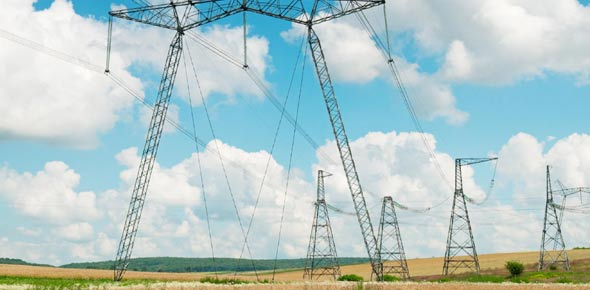What Is Electricity Quiz?

30 Questions | Total Attempts: 133SettingsTake this educational science quiz and learn about batteries, conductors, insulators, amps, watts, generators, power, and volts.

• 1.
An _______ is a device for measuring resistance.
• 2.
What happens when a switch is turned off
• A.

Electricity stops flowing from the switch and therefore the circuit is broken, providing no electricity to the object being powered

• B.

Electricity is able to flow through the circuit powering an electrical device

• C.

Electricity is not able to flow through the circuit but it can still power an electrical device

• D.

Electricity is able to flow through the circuit but it cannot power an electrical device

• 3.
Why should you never directly connect the negative and positive terminals of a battery?
• A.

It will short circuit

• B.

You will get a shock

• C.

The battery will turn blue

• D.

The battery will disappear

• 4.
The unit used for measuring electrical current is called a(an):
• A.

Electrode

• B.

Volt

• C.

Discharge

• D.

Ampere

• 5.
Material that electricity can travel through easily is called a
• A.

Conductor

• B.

Insulator

• C.

Semiconductor

• D.

None of these

• 6.
A circuit is a path taken by a current. A path with no breaks is called a
• A.

Closed circuit

• B.

None of these

• C.

Open switch

• D.

Open circuit

• 7.
Materials that do not allow electrical charges to pass through it easily are called
• A.

Conductor

• B.

All of the above

• C.

Semiconductors

• D.

Insulator

• 8.
Parallel circuits have only one path.
• A.

True

• B.

False

• 9.
Two identical batteries are connected.  Their total voltage is exactly twice as much as either battery on its own.  Most likely, they are connected how?
• A.

In series

• B.

In parallel

• C.

Most likely they are not connected to one another

• 10.
Why are resistors used in a circuit?
• A.

To store electrical energy

• B.

To make current switch back and forth rapidly

• C.

To control how much current flows through a particular part of the circuit so other components work properly

• D.

Resistors don't really exist, they are just a concept

• 11.
True or False.  Circuit diagrams are schematic maps drawn to represent the connections between electrical components like batteries, resistors, light bulbs, etc.
• A.

True.

• B.

False.

• 12.
A certain steam iron carries a current of 6.4 A when connected to a 120 V source.  What is the resistance of the steam iron?
• A.

1.875 Ω

• B.

0.05 Ω

• C.

768 Ω

• D.

18.75 Ω

• 13.
The resistance of a hotplate is 48 Ω .  How much current does the plate carry when connected to a 120-V source?
• A.

120 A

• B.

0.4 A

• C.

5760 A

• D.

2.5 A

• 14.
Voltage is a measurement of what?
• A.

Radiant energy of a lightbulb in a circuit

• B.

Kinectic energy of a motor in a circuit

• C.

Potential energy stored in a battery

• D.

The flow of electrons

• 15.
What is current?
• A.

The newness of a battery or resistor

• B.

Flow of charge, usually in the form of electrons

• C.

Something that blocks or slows the flow of electrons

• D.

The potential energy stored in a battery

• 16.
Current flowing in only one direction is
• A.

Unpredictable Current (UC)

• B.

Alternating Current (AC)

• C.

Direct Current (DC)

• D.

Detectable Current (DC)

• 17.
What is the unit for voltage?
• A.

Ampere

• B.

Joule

• C.

Volt

• D.

Hertz

• E.

Ohm

• 18.
What is the unit for resistance?
• A.

Ampere

• B.

Joule

• C.

Volt

• D.

Hertz

• E.

Ohm

• 19.
Two identical batteries are connected.  Their total voltage is exactly twice as much as either battery on its own.  Most likely, they are connected how?
• A.

In series

• B.

In parallel

• C.

Most likely they are not connected to one another

• 20.
Why are resistors used in a circuit?
• A.

To store electrical energy

• B.

To make current switch back and forth rapidly

• C.

To control how much current flows through a particular part of the circuit so other components work properly

• D.

Resistors don't really exist, they are just a concept

• 21.
True or False.  Circuit diagrams are schematic maps drawn to represent the connections between electrical components like batteries, resistors, light bulbs, etc.
• A.

True.

• B.

False.

• 22.
A certain steam iron carries a current of 6.4 A when connected to a 120 V source.  What is the resistance of the steam iron?
• A.

1.875 Ω

• B.

0.05 Ω

• C.

768 Ω

• D.

18.75 Ω

• 23.
The resistance of a hotplate is 48 Ω .  How much current does the plate carry when connected to a 120-V source?
• A.

120 A

• B.

0.4 A

• C.

5760 A

• D.

2.5 A

• 24.
Voltage is a measurement of what?
• A.

Radiant energy of a lightbulb in a circuit

• B.

Kinectic energy of a motor in a circuit

• C.

Potential energy stored in a battery

• D.

The flow of electrons

• 25.
What is current?
• A.

The newness of a battery or resistor

• B.

Flow of charge, usually in the form of electrons

• C.

Something that blocks or slows the flow of electrons

• D.

The potential energy stored in a battery

Related TopicsBack to top